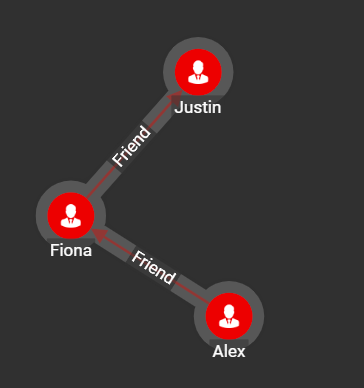Breadth-first Search (BFS) is an algorithm used to explore the vertexes of a graph layer by layer. It starts at the given vertex and explores all vertices at the present depth prior to moving on to the vertices at the next depth level.

## Specifications

```CREATE QUERY tg_bfs(SET<STRING> v_type, SET<STRING> e_type,INT max_hops=10,
VERTEX v_start, BOOL print_accum = True, STRING result_attr = "",
STRING file_path = "", BOOL display_edges = True)```
Characteristic Value

Result

Returns all the nodes that are accessible from the source vertex

Required Input Parameters

• `v_type`: vertex types to traverse

• `e_type`: edge types to traverse

• `max_hops`: look only this far from each vertex

• `v_start`: source vertex for traversal

• `print_accum`: print JSON output

• `result_attr`: INT attr to store results to

• `file_path`: file to write CSV output to

• `display_edges`:output edges for visualization

Result Size

V = number of vertices

Time Complexity

O(E+V), E = number of edges, V = number of vertices.since every vertex and every edge will be explored in the worst case.

Graph Types

Directed or Undirected edges, Weighted or Unweighted edges

## Example

In the example below, we run `tg_bfs` algorithm from the source vertex `alex` on the social10 graph.

```# Use _ for default values
RUN QUERY tg_bfs(["Person"], ["Friend"], _, ("Alex","Person"), _, _, _, _)```

Below is the visualized result of the query: